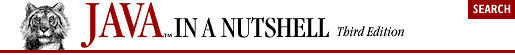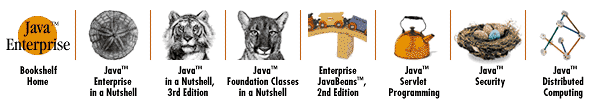## 4.5. Arrays

The java.lang.System class defines an arraycopy() method that is useful for copying specified elements in one array to a specified position in a second array. The second array must be the same type as the first, and it can even be the same array:

```char[] text = "Now is the time".toCharArray();
char[] copy = new char;
// Copy 10 characters from element 4 of text into copy, starting at copy
System.arraycopy(text, 4, copy, 0, 10);

// Move some of the text to later elements, making room for insertions
System.arraycopy(copy, 3, copy, 6, 7);```

In Java 1.2 and later, the java.util.Arrays class defines useful array-manipulation methods, including methods for sorting and searching arrays:

```import java.util.Arrays;

int[] intarray = new int[] { 10, 5, 7, -3 }; // An array of integers
Arrays.sort(intarray);                       // Sort it in place
int pos = Arrays.binarySearch(intarray, 7);  // Value 7 is found at index 2
pos = Arrays.binarySearch(intarray, 12);     // Not found: negative return value

// Arrays of objects can be sorted and searched too
String[] strarray = new String[] { "now", "is", "the", "time" };
Arrays.sort(strarray);      // { "is", "now", "the", "time" }

// Arrays.equals() compares all elements of two arrays
String[] clone = (String[]) strarray.clone();
boolean b1 = Arrays.equals(strarray, clone);  // Yes, they're equal

// Arrays.fill() initializes array elements
byte[] data = new byte;          // An empty array; elements set to 0
Arrays.fill(data, (byte) -1);         // Set them all to -1
Arrays.fill(data, 5, 10, (byte) -2);  // Set elements 5, 6, 7, 8, 9 to -2```

Arrays can be treated and manipulated as objects in Java. Given an arbitrary object o, you can use code such as the following to find out if the object is an array and, if so, what type of array it is:

```Class type = o.getClass();
if (type.isArray()) {
Class elementType = type.getComponentType();
}```Copyright © 2001 O'Reilly & Associates. All rights reserved.